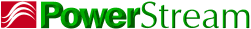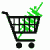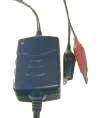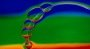July 31, 2019

How to calculate battery run-timeBattery Chargers More Engineering Resources Battery capacity tester for small and tiny cells Batteries and Packs Precision Spot Welder

For a Java-script calculator that gives reasonable estimated of battery run-time click here.

Notes for Design Engineers: How to calculate how much battery capacity you need.

I know, I feel your pain. The marketing department gave you a specification and all it says is “maximize run time, minimize the battery size and cost." But they won't tell you much run time is acceptable, how much size and weight will the market put up with, what cost is acceptable?

Hey, the reason that they aren't more specific is they are hoping for a miracle and don't want over-specify in case they don't get the miracle. The miracle you were hoping for was a complete specification, but let's get real here.

Your revenge is to wait 2 weeks and come back with “Good news, I fit it in a fountain pen for a BOM of only \$5000 and by trimming the power budget (i.e. eliminating all but one of the features) we got it to run for over 5.5 seconds before recharging.And then sit back and hope for better guidance from marketing!

You already knew that I couldn’t help you with your specification, but at least you can use the following design estimation tools to give the marketing department a matrix of choices.

How much battery capacity do you need to run your device? Here is how you estimate it.

Step 0. A little tutorial on measurements of electronic charge. After all, it is electrons (really ions) that are stored in the battery. In phreshman fisicks we all learned that the measure of charge is the coulomb and that a single electron has 1.602e-19 coulombs of charge. One amp flowing in a wire for one second will use one coulomb of charge, which is 6.24 x 10^18 electrons,.

Q = I*t

where Q is the charge in coulombs, I is the current in amps and t is the time in seconds.

The amount of charge passing through that wire (conducting 1.0 amps) in 60 seconds is 60 coulombs, and in one hour you would have had said “hello” and “good-bye” to 3600 coulombs of charge.

Batteries were evidently developed by engineers who subscribed to the “whatever’s easiest” system of measurement. They got tired of pulling out their slide rules to divide by 3600 every time they wanted to know how long 24000 coulombs would last them and came up with the unauthorized unit of amp-hours. Later, when smaller batteries were used they came up with milliamp-hours.

Don’t be confused by the hyphen. Amp-hours means amps times hours. Divide by amps and you get hours, divide by hours and you get amps. So it isn’t amps, and it isn’t amps per hour, it is amp-hours. And, by the way, I have even used the term amp-seconds because when you say “coulombs” everybody goes glassy-eyed on you.

Don’t get me wrong, I love amp-hours for units, it is a handy rule of thumb. Amp hours is how much charge is stored in the battery. Since a battery changes voltage during the discharge, it isn’t a perfect measure of how much energy is stored, for this you would need watt-hours. Multiplying the average or nominal battery voltage times the battery capacity in amp-hours gives you an estimate of how many watt-hours the battery contains.

E = C*Vavg

Where E is the energy stored in watt-hours, C is the capacity in amp-hours, and Vavg is the average voltage during discharge. Yes, watt-hours is a measure of energy, just like kilowatt-hours. Multiply by 3600 and you get watt-seconds, which is also known as Joules.

As long as we are in the prelude, I might also mention that since the charge in a capacitor is Q=CV that a battery can be rated in farads as well. A 1.5 volt AA alkaline battery that stores 2 amp hours of charge (that’s 7200 coulombs) has the equivalent capacitance of 4800 Farads. Of course a battery makes an awfully weird capacitor because the voltage doesn’t drop proportionally to the stored charge, it has a high equivalent resistance, and etc.

Also, I should mention that you don't always get all the amp-hours you expect out of a battery. This is explained in Part 3 below as the Peukart effect. This is why I called it a rule-of-thumb rather than a theorem. The biggest errors come when you discharge batteries fast. Some batteries, such as Carbon-Zinc, Alkaline, or Lead Acid become less efficient when you discharge quickly. A typical sealed lead acid battery will give only half of its rated capacity when discharged at the C/1 rate compared with the C/20 rate.

The following method assumes that you know how many amps you need for the gadget under power. If you know the watts go to Step A below.

Step 1. Back of the envelope

If the current drawn is x amps, the time is T hours then the capacity C in amp-hours is

C = xT

For example, if your pump is drawing 120 mA and you want it to run for 24 hours

C = 0.12 Amps * 24 hours = 2.88 amp hours

Step 2. Cycle life considerations

It isn’t good to run a battery all the way down to zero during each charge cycle. For example, if you want to use a lead acid battery for many cycles you shouldn’t run it past 80% of its charge, leaving 20% left in the battery. This not only extends the number of cycles you get, but lets the battery degrade by 20% before you start getting less run time than the design calls for

C’ = C/0.8

For the example above

C’ = 2.88 AH / 0.8 = 3.6 AH

Step 3: Rate of discharge considerations

Some battery chemistries give much fewer amp hours if you discharge them fast. This is called the Peukart effect. This is a big effect in alkaline, carbon zinc, zinc-air and lead acid batteries. For example if you draw at 1C on a lead acid battery you will only get half of the capacity that you would have if you had drawn at 0.05C. It is a small effect in NiCad, Lithium Ion, Lithium Polymer, and NiMH batteries.

For lead acid batteries the rated capacity (i.e. the number of AH stamped on the side of the battery) is typically given for a 20 hour discharge rate. If you are discharging at a slow rate you will get the rated number of amp-hours out of them. However, at high discharge rates the capacity falls steeply. A rule of thumb is that for a 1 hour discharge rate (i.e. drawing 10 amps from a 10 amp hour battery, or 1C) you will only get half of the rated capacity (or 5 amp-hours from a 10 amp-hour battery). Charts that detail this effect for different discharge rate can be used for greater accuracy. For example the data sheets listed in /BB.htm

For example, if your portable guitar amplifier is drawing a steady 20 amps and you want it to last 1 hour you would start out with Step 1:

C=20 amps * 1 hour = 20 AH

Then proceed to Step 2

C’ = 20 AH / 0.8 = 25 AH

Then take the high rate into account

C’‘=25 /.5 = 50 AH

Thus you would need a 50 amp hour sealed lead acid battery to run the amplifier for 1 hour at 20 amps average draw.

Step 4. What if you don’t have a constant load? The obvious thing to do is the thing to do. Figure out an average power drawn. Consider a repetitive cycle where each cycle is 1 hour. It consists of 20 amps for 1 second followed by 0.1 amps for the rest of the hour. The average current would be calculated as follows.

20*1/3600 + 0.1(3599)/3600 = 0.1044 amps average current.

(3600 is the number of seconds in an hour).

In other words, figure out how many amps is drawn on average and use steps 1 and 2. Step 3 is very difficult to predict in the case where you have small periods of high current. The news is good, a steady draw of 1C will lower the capacity much more than short 1C pulses followed by a rest period. So if the average current drawn is about a 20 hour rate, then you will get closer to the capacity predicted by a 20 hour rate, even though you are drawing it in high current pulses. Actual test data is hard to come by without doing the test yourself.

If you know the watts instead of amps, follow the following procedure

Step A: Convert watts to amps

Actually, watts is the fundamental unit of power and watt-hours is the energy stored. The key is to use the watts you know to calculate the amps at the battery voltage .

For example, say you want to run a 250 watt 110VAC light bulb from an inverter for 5 hours.
Watt-hours = watts * hours = 250 watts * 5 hours = 1250 watt hours

Account for the efficiency of the inverter, say 85%

Watt-hours = watts * hours / efficiency = 1250 / 0.85 = 1470 watt-hours

Since watts = amps * volts divide the watt hours by the voltage of the battery to get amp-hours of battery storage

Amp-hours (at 12 volts) = watt-hours / 12 volts = 1470 / 12 = 122.5 amp-hours.
If you are using a different voltage battery the amp-hours will change by dividing it by the battery voltage you are using.

Now go back to Steps 2-4 above to refine your calculation.Thank you for considering PowerStream

Industrial battery engineering. Battery design. Custom batteries. Building battery packs. Battery information. Battery info.

 PowerStream Technology 1163 South 1680 West Orem Utah 84058 Phone: 801-764-9060 Fax: 801-764-9061 © Copyright 2000, 2002, 2003, 2004, 2005, 2006, 2008,2009, 2010, 2011, 2012, 2013, 2014, 2015, 2016, 2017, 2018, 2019 Lund Instrument Engineering, Inc. All rights reserved. Google Author Verification